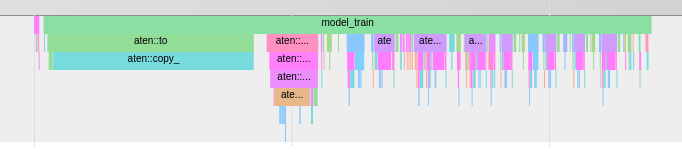# What is aten:to in the profiler

This is a profile of

``````with profiler.profile(record_shapes=True) as prof:
with profiler.record_function("model_train"):
out = model.forward(data.to(DEVICE))
loss = compute_loss(data[1:], out)
loss.backward()
``````

What’s up with the long `aten:to` block in the middle? At first I thought it was the operation of copying tensors from cpu to gpu. This still makes sense to me, but I don’t understand why the second block is so long.

As part of computing my loss I do a `to(DEVICE)` on my targets, but that’s supposed to be relatively very small.

``````criterion = nn.CrossEntropyLoss(ignore_index=tkn.stoi['<pad>'])

def compute_loss(targets, outputs):
# targets shape [(B, padded_seq_length), (B, 1)]
# outputs shape (B, S, N+1) S is sequence length of output, N is n_classes
caption_lengths, sort_ind = targets.squeeze(1).sort(dim=0, descending=True)
decode_lengths = (caption_lengths - 1).tolist()
targets = pack_padded_sequence(targets[sort_ind][:, 1:].to(DEVICE), decode_lengths, batch_first=True).data
loss = criterion(predictions, targets)
return loss
``````

Then, I tried returning `loss=0` without actually computing a loss like this:

``````criterion = nn.CrossEntropyLoss(ignore_index=tkn.stoi['<pad>'])

def compute_loss(targets, outputs):
# targets shape [(B, padded_seq_length), (B, 1)]
# outputs shape (B, S, N+1) S is sequence length of output, N is n_classes
caption_lengths, sort_ind = targets.squeeze(1).sort(dim=0, descending=True)
decode_lengths = (caption_lengths - 1).tolist()
targets = pack_padded_sequence(targets[sort_ind][:, 1:].to(DEVICE), decode_lengths, batch_first=True).data
return nn.Parameter(torch.tensor().float())
``````

This actually gets rid of the second `aten:to` blockSo why is the loss taking so long to compute? And was I right in saying that `aten:to` is the cpu > gpu operation? If so, what does this have to do with calculating the loss?

On a side note, I noticed that as I scale my model size, that second aten:to block takes longer to do (proportionally to the total time of the loop iteration). Why should it, if the loss is calculated independently of model size?

Hello @Alexander_Soare! Did do manage to catch what is the source of that long `aten::to` block? I am running into a similar issue.

Nope sorry. This problem has been long forgotten.